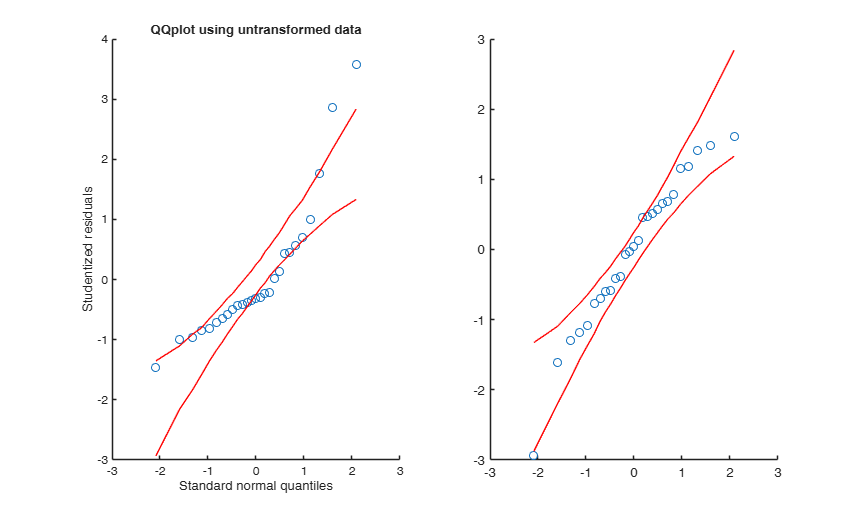# qqplotFS

qqplotFS creates qqplot of studentized residuals with envelopes

## Syntax

• Y=qqplotFS(res)example
• Y=qqplotFS(res,Name,Value)example

## Description

Displays a quantile-quantile plot of the quantiles of the sample studentized residuals versus the theoretical quantile values from a normal distribution. If the distribution of residuals is normal, then the data plot appears linear. A confidence level is added to the band.

 Y =qqplotFS(res) qqplot for the multiple regression data.

 Y =qqplotFS(res, Name, Value) qqplot with envelopes for the Wool data.

## Examples

expand all

###qqplot for the multiple regression data.

This is an example of the use of options X and plots

load('multiple_regression.txt');
y=multiple_regression(:,4);
X=multiple_regression(:,1:3);
outLM=fitlm(X,y,'exclude','');
res=outLM.Residuals{:,3};
qqplotFS(res,'X',X,'plots',1);
title('qqplot of stud. res.')
% No outlier appears###qqplot with envelopes for the Wool data.

Compare the results using untransformed and transformed data.

% This is an example of the use of option h
y=(XX(:,end));
lny=log(y);
X=XX(:,1:end-1);
outLM=fitlm(X,y,'exclude','');
res=outLM.Residuals{:,3};
outLMtra=fitlm(X,lny,'exclude','');
restra=outLMtra.Residuals{:,3};
h1=subplot(1,2,1);
qqplotFS(res,'X',X,'plots',1,'h',h1);
title('QQplot using untransformed data')
h2=subplot(1,2,2);
qqplotFS(restra,'X',X,'plots',1,'h',h2);
title('QQplot using transformed data')## Input Arguments

### res — vector containing studentizd residuals. Vector.

Vector with n elements containing the studentized residuals from a regression model

Data Types: single| double

### Name-Value Pair Arguments

Specify optional comma-separated pairs of Name,Value arguments. Name is the argument name and Value is the corresponding value. Name must appear inside single quotes (' '). You can specify several name and value pair arguments in any order as  Name1,Value1,...,NameN,ValueN.

Example:  'conflev',0.99 , 'intercept',false , 'nsimul',300 , 'X',randn(n,3) , 'plots',1 ,'h',h1 where h1=subplot(2,1,1) ,

### conflev —Confidence level which is used to compute confidence bands of studentized residuals.scalar Usually conflev=0.

95, 0.975 0.99 (individual alpha) or 1-0.05/n, 1-0.025/n, 1-0.01/n (simultaneous alpha).

Default value is 0.90

Example:  'conflev',0.99 

Data Types: double

### intercept —Indicator for constant term.true (default) | false.

Indicator for the constant term (intercept) in the fit, specified as the comma-separated pair consisting of 'Intercept' and either true to include or false to remove the constant term from the model.

Example:  'intercept',false 

Data Types: boolean

### nsimul —number of simulations to compute the envelopes.scalar.

The default value is 1000.

Example:  'nsimul',300 

Data Types: double

### X —Predictor variable.matrix.

Data matrix of explanatory variables (also called 'regressors') of dimension (n x p-1). Rows of X represent observations, and columns represent variables. This is the matrix which has been used to produce stdentized residuals.

If this optional argument is missing we take as matrix X the column of ones.

Example:  'X',randn(n,3) 

Data Types: double

### plots —Plot on the screen.scalar.

If plots = 1, a plot which shows the robust the qqplot of residuals with envelopes is shown on the screen. The confidence level which is used to draw the horizontal lines associated with the bands for the residuals is specified in input option conflev. If conflev is missing a nominal 0.90 confidence interval will be used.

Example:  'plots',1 

Data Types: double

### h —the axis handle of a figure where to send the qqplot.this can be used to host the qqplot in a subplot of a complex figure formed by different panels (for example a panel with qqplot from a classical ols estimator and another with qqplot from a robust regression).

Example: 'h',h1 where h1=subplot(2,1,1) 

Data Types: Axes object (supplied as a scalar)

### tag —handle of the plot which is about to be created.character.

The default is to use tag 'pl_qq'. Notice that if the program finds a plot which has a tag equal to the one specified by the user, then the output of the new plot overwrites the existing one in the same window else a new window is created

Example:   'tag','mytag'

Data Types: char

## Output Arguments

### Y —matrix with raws data on which the plot is based.  n-by-3 matrix

1st col = standard normal quantiles.

2nd col = quantiles of input sample of studentized residual.

3rd col = lower confidence band of quantiles of studentized residuals.

4th col = upper confidence band of quantiles of studentized residuals.

Atkinson, A.C. and Riani, M. (2000), "Robust Diagnostic Regression Analysis", Springer Verlag, New York.